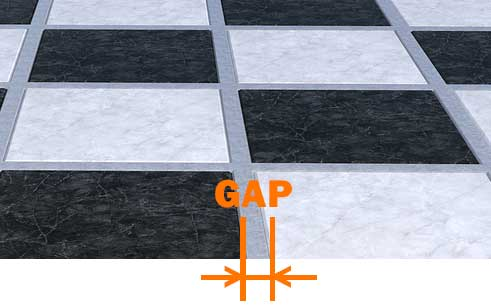# Tile Calculator

Calculator

Tile Calculator – a simple and easy way for estimating the number of tiles required to cover an area. Do you want to know how the number of tiles needed to cover a floor, wall or any other surface? Thanks to our free online Tile Calculator, you can easily get it in seconds. Just give us the size of the area to cover, the tile size, and the gap size between the titles.

AREA TO COVER
TILE SIZE
GAP SIZE
result

Q&A

## Information about the Tile Calculator

• How do I calculate the required amount of tile for covering the area? Basically, the first thing to do is calculating the size of the area to be covered. In most cases it is a rectangle which has two dimensions (width, length). This have to be multiplied each other to give the surface to be tiled. If you want to know exactly how many tiles you need, then you have to divide the result by the size of the tiles. That’s how our calculator works.

• Can I calculate with title gaps and/or overlaps? Sure! Our Tile Calculator can deal with gaps and overlaps, too. If the tiles somehow overlap each other, you should give a negative value as overlap.